# DAV Class 6 Maths Chapter 9 Brain Teasers Solutions

The DAV Maths Book Class 6 Solutions and DAV Class 6 Maths Chapter 9 Brain Teasers Solutions of Line Segments offer comprehensive answers to textbook questions.

## DAV Class 6 Maths Ch 9 Brain Teasers Solutions

Question 1.
A. Tick (✓) the correct answer.
(a) In the given figure, the number of line segments are:(i) 5
(ii) 10
(iii) 15
(iv) 20
In the given figure, there are 10 line segments. They are AB, AC, AD, AE, BC, BD, BE, CD, CE and DE Thus, the (ii) option is correct.

(b) The number of diagonals in a pentagon are
(i) 2
(ii) 3
(iii) 4
(iv) 5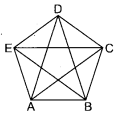A pentagon has five sides.
ABCDEA is a pentagon. AB, BC, CD, DE and EA are its five sides. AC, AD, BD, BE and CE are its diagonals. They are five in number. Thus, a pentagon has five diagonals.

(c) For the following figure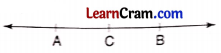(i) AC + CB = AB
(ii) AB + AC = CB
(iii) AB + BC = CA
(iv) none
AC + CB = AB is the correct option.

(d) A line segment
(i) extends in one direction only.
(ii) extends in both the directions.
(iii) has two end points.
A line segment has two end points and has a definite length. It is a portion of a line. Thus, option (iii) is correct.

(e) One end point of a line segment AB is against the mark 2 and other at mark 9 of a scale. What is the length of segment AB?
(i) 6 cm
(ii) 7 cm
(iii) 9 cm
(iv) 11 cm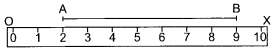AB is a line segment and OX is a scale. Point A is at 2 and Point B is at 9. So, length of segment AB = OB – OA = 9 – 2 = 7 cm.
Thus, option (ii) is correct.(a) For the given figure,(i) AE + EC = _______
(ii) AC – EC = _______
(iii) BD – BE = _______
(iv) BD – DE = _______
(i) AE + EC = AC
(ii) AC – EC = AE
(iii) BD – BE = DE
(iv) BD – DE = BE

(b) The length of an air conditioner remote is 10 cm and length of a television remote is 16 cm. Construct a line segment of length equal to the difference of their length.Step 1. Draw a length AB of 10 cm equal to the length of an air conditioner remote.
Step 2. Draw a length XY of 16 cm equal to the length of television remote.
Step 3. Using compass, draw a line segment AB, equal to 10 cm on XY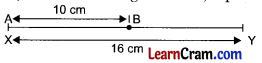Step 4. XY – AB = 16 cm – 10 cm = 6 cm = BY
Here, BY is the difference of lengths XY and AB.

(c) Measure the length of your Papa’s mobile using compasses and construct the line segment of that length.
Length of mobile phone = 4 cm
Using compass, draw a line segment PQ of 4 cm starting from P. Thus, PQ = 4 cm.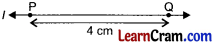(d) Rajan purchased a ribbon of length 20 cm to tie his project report. He used 14 cm ribbon. Construct a line segment of the same length as the difference of given length.
Total length of the ribbon = 20 cm
Ribbon used to tie the project report = 14 cm
Difference of the length of ribbon = 20 cm – 14 cm = 6 cm
To construct a line segment of 6 cm, we draw a line segment of AB by using compass.Starting from point A, we draw another point B on the line l and length equal to 6 cm.

(e) How many line segments are there in the following figure?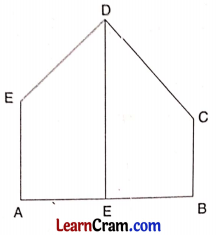In the figure, AE, AB, BE, BC, CD, DE, DF and FA are the line segments. Thus, there are a total of 8 line segments.

Question 2.
How many line segments are there in the given figure? Name any four.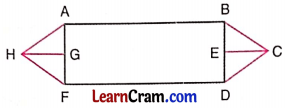The given figure has 14 line segments.
AB, BD, FD, AF, BC, CD, EC, AH, HF, HG, GF, AG, BE, EDQuestion 3.
Construct a line segment whose length is thrice the length of the given line segment.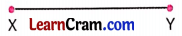Step 1: Draw any line l and take a point A on it.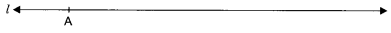Step 2: Using compass and starting from A construct a line segment AB on l equal to the length of the given line segment XY.Step 3: Again using compass and starting from B (to the right) construct another line segment BC equal to the length of the given line segment.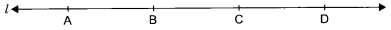Step 4: Now starting from C, construct a line segment CD on l equal to the length of the given line segment XY.
Hence AB = AB + BC + CD = XY + XY + XY = 3 XY

Question 4.
You are given a triangle here. Construct a line segment whose length is equal to the sum total of all the three sides of this triangle.Step 1: Draw any line l and take a point A on it.Step 2: Using compass and starting from A, draw a line segment AB on l equal to the length of PQuestionStep 3: Now, with the help of compass, draw a line segment BC on l equal to the length of the side QR of the triangle PQR.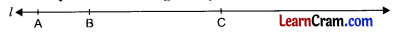Step 4: Using compass and starting from B, draw a line segment BC on l equal to the length of the side RP of the triangle PQR.Hence AD = PQ + QR + RP.

Question 5.
Find the perimeter of a square of side 2 cm and of an equilateral triangle of side 1.5 cm. Construct—
(a) a line segment whose length is the sum of their perimeters.
(b) a line segment whose length is difference of their perimeters.
To find perimeter of a square, draw line segments AB, BC, CD and DA of equal length of 2 cm each. Thus,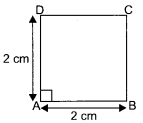Perimeter of the square = AB + BC + CD + DA
= 2 cm + 2 cm + 2 cm + 2 cm
= 8 cm
Again, to find perimeter of an equilateral triangle we draw line and PR of equal length of 1.5 cm.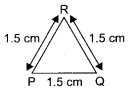Thus,
Perimeter of equilateral triangle = PQ + QR + RP
= 1.5 cm + 1.5 cm + 1.5 cm
= 4.5 cm

(a) Now, sum of perimeters = Perimeter of square + perimeter of equilateral triangle
= 8 cm + 4.5 cm
= 12.5 cm
To construct a line segment of length 12.5 cm.
Step 1. With the help of compass, draw a line l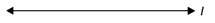Step 2. Mark a point A on the line.Step 3. From point A on the line, take another point B on the line such that the AB = 12.5 cm.Thus, AB = 12.5 cm is the sum of perimeters of square and triangle.

(b) Perimeter of square = 8 cm
Perimeter of equilateral triangle = 4.5 cm
So, their difference = 8 cm – 4.5 cm = 3.5 cm
Steps to construct a line segment of length 3.5 cm
(i) Using compass draw a line l.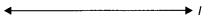(ii) Mark a point A on the line l.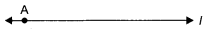(iii) With A as centre and length equal to 3.5 cm, mark another point B on the same line.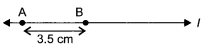Thus, AB = 3.5 cm.

### DAV Class 6 Maths Chapter 1 HOTS

Question 1.
There are five points on a sheet of paper, such that no three are collinear. What is the number of line segments that can be drawn by joining the points in pairs?
There are five different points P, Q, R, S and T such that no three are collinear.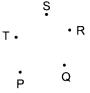By joining them in pairs, we get, PQ, PR, PS, PT, QR, QS, QT, RS, RT and ST.There are a total of 10 line segments.Question 2.
In each of the following cases, state whether you can draw line segments on the given surfaces.
(i) Face of a cube
(ii) The top of a solid cylinder
(iii) The surface of an apple
(iv) Curved surface of a cone.
(i) Yes, a cube has 6 faces.
(ii) Yes, because top of a cylinder is a plane surface at circular end.
(iii) No, because apple is spherical in shape and error caused in actual measurement.
(iv) No, a line segment cannot be drawn on the curved surface of a cone.

Question 1.
How many line segments are there in the given figure? Measure the lengths of each of the line segment with the help of ruler.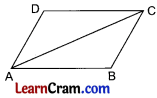There are 5 line segments in the given figure.
Using ruler, we find that
AB = 3.2 cm, DC = 3.2 cm, AD = 2 cm, BC = 2 cm and AC = 3.5 cm.

Question 2.
Draw a line segment whose length is equal to the sum of the given line segments XY and ZW.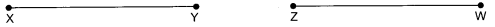Step 1: Draw a line l and take any point A on it.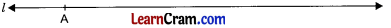Step 2: Using compass, starting from A, construct a line segment AB on l equal to the line-segment XY.Step 3: Starting from B(to the right) construct a, line-segment BC on l equal to the length of the line segment ZW.
Hence AC = XY + ZW.

Question 3.
Compare the following pairs of line segments and put >, = or < in the blanks:(a) XY > PQ
(b) AB < CD
(c) MN = OP
(d) RS > TM

Question 4.
Construct a line segment PQ = 5.5 cm. From this line segment cut off a line segment QR = 3 cm. Measure the length of the remaining line segment PR.
Step 1 : Draw a line l and take any point P on it.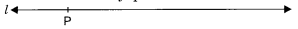Step 2: Using compass and starting from P, construct a line segment PQ = 5.5 cm.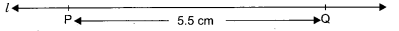Step 3: Starting from Q (to the left) cut off a line segment QR = 3 cm from the line segment PQ.Hence the required line segment is PR whose length is 2.5 cm.Question 5.
Measure the length and breadth of a playing card. Construct a line segment whose length is their
(a) Sum
(b) Difference
(a) Length of the playing card = 8 cm
Breadth of the playing card = 4 cm
Sum of the length and breadth = 8 + 4 = 12 cm
Step 1 : Draw a line l and take any point A on it.Step 2: Using compass, starting from A, construct a line segment AB on l equal to the length of the playing card i.e. 8 cm.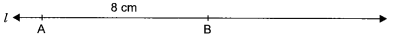Step 3: Now starting from B (to the right) draw a line segment BC on l equal to the breadth of the playing cards i.e. 4 cm.Here AC is the segment which represents the sum of the length and breadth of the playing card.
∴ AC = AB + BC
= 8 cm + 4 cm
= 12 cm

(b) Step 1: Draw any line l and take any point P on it.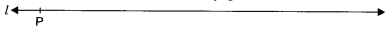Step 2: Using compass, starting from P, draw a line segment PQ on l equal to the length of the playing card.Step 3: Now starting from Q(to the left), draw a line segment QR on l equal to the breadth of the playing card.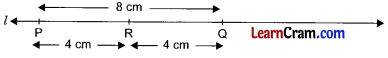Hence PR is the line segment whose length is equal to the difference of the length and breadth of the playing cards.
where PR = PQ – QR
= 8 cm – 4 cm
= 4 cmQuestion 6.
The end point P of a line segment PQ is against 2 cm mark and the end point Q is against the mark indicating 9 cm on a ruler. What is the length of the line segment?